# Big-O: How Code Slows as Data Grows

This is a presentation for PyCon 2018 in Cleveland. You can read the slides and text on this page, or watch the video, or open the actual presentation in your browser (use right and left arrows to advance the slides).

Also, this is a re-working of an earlier piece with the same name: Big-O: how code slows as data grows. It covers the same ideas, but with different text and figures.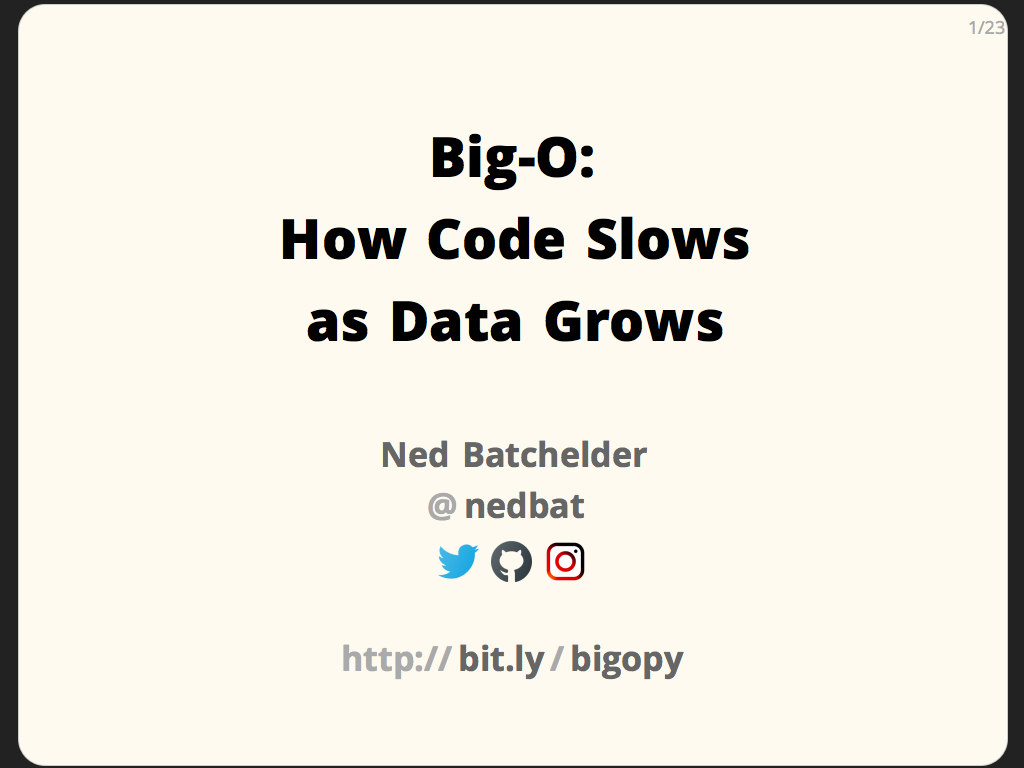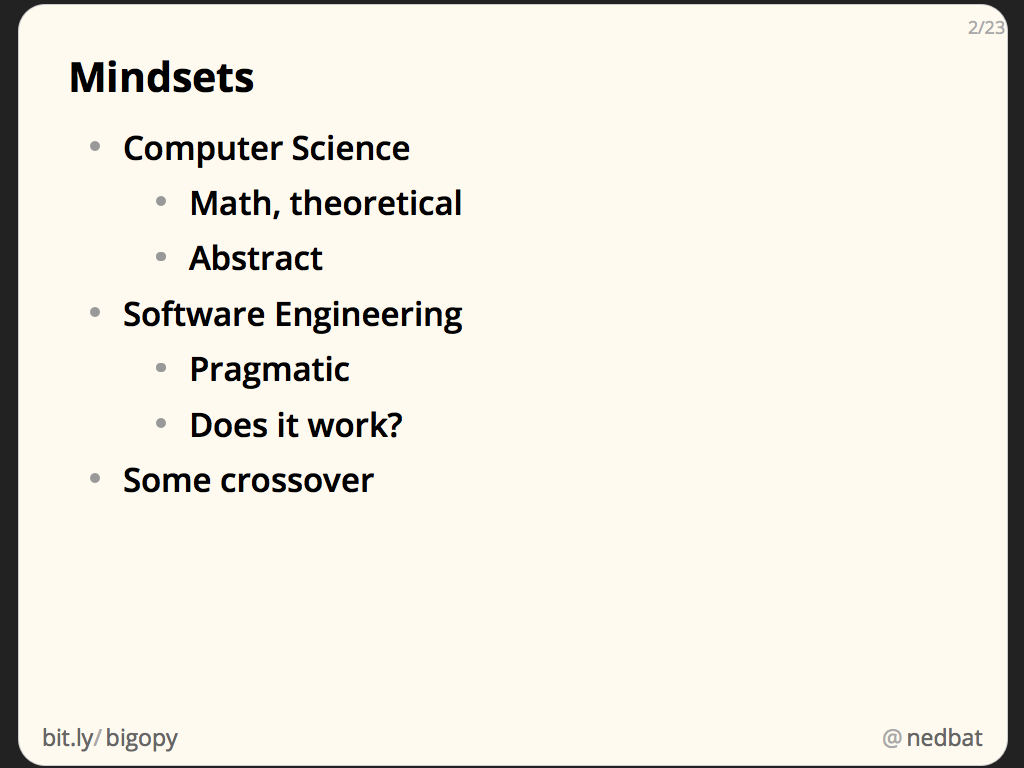One of the things that interests me about writing software is the two mindsets that inform it. One is computer science, another is software engineering.

Computer science is a branch of mathematics. It’s full of complex notation about abstract ideas, and proofs of mathematical principles. Software engineering is focused on how to actually write software that works. The two sides interact, but they are very different.

If you are working in software, but don’t have a computer science degree, you might feel like there are mysteries and incantations you are missing that you need. For the most part, computer science is about things you don’t encounter in day-to-day software development.

But one topic that does cross the boundary is a thing called algorithmic analysis, or Big-O notation.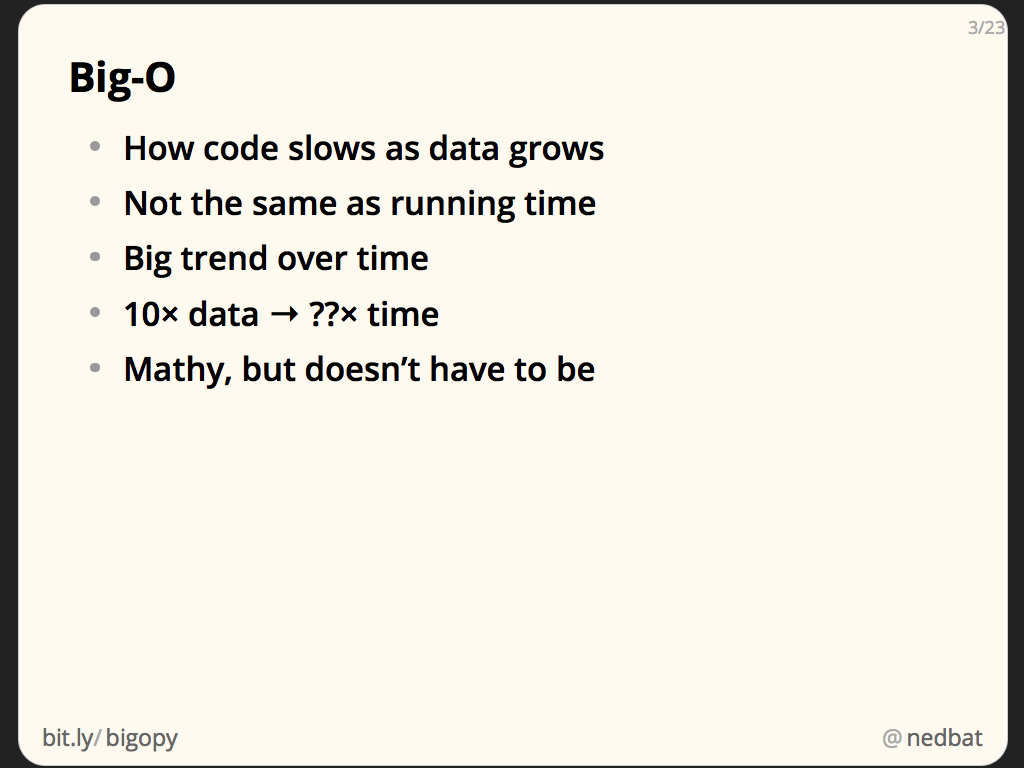Big-O isn’t as mystical as it seems at first. Big-O is a way of characterizing how your code’s speed is affected by the size of the data it’s working on. There’s a lot of theory behind it, but it doesn’t have to be more than a common-sense assessment of how your code will behave as it works on more data.

In rhyming form, “How code slows as data grows.” Big-O isn’t about how fast your code goes at any particular time. It’s about how the running time changes as the data size changes.

Imagine you have a chunk of code that does something to a bunch of data. You’d like to know how much slower the code will go if you give it ten times more data. That’s what big-O describes. You might imagine that the answer is of course, ten times slower. But that’s not the way real code works. One algorithm might go a hundred times slower. Another might not go any slower at all. Big-O is a way to analyze the code and determine the slow-down factor.

Big-O notation has a mathematical flavor, but isn’t really that complicated in day-to-day use. You can use it without understanding all of the intricacies.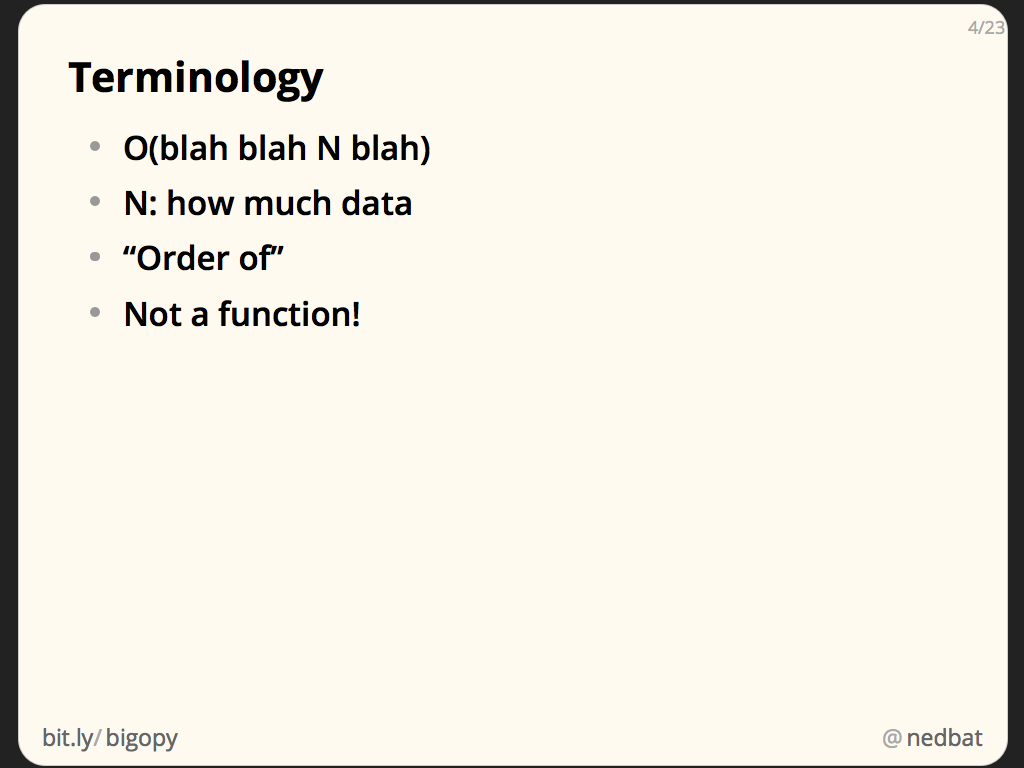Let’s get some terminology straight at the beginning. The Big-O characterization of some code will look like “O( blah blah N blah )”. The N in there is the size of your data. The O stands for “Order of,” as in, “The code’s run time grows on the order of blah blah N blah.” The blah blah is an expression using N, like O(N) or O(N2).

Although O(N) looks like calling a function named O, this isn’t a function call. It’s just a notation about N.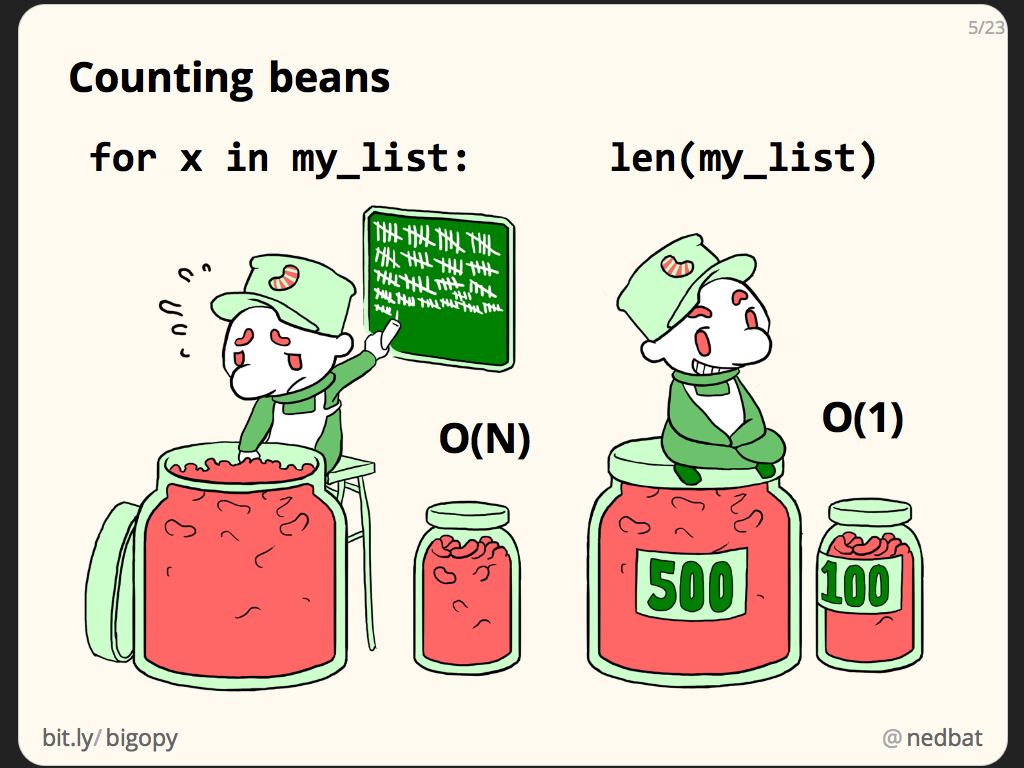Let’s consider a real-world example. Suppose you have jars of jelly beans. You want to know how many are in each jar. The first way to do it is to count each bean, as this guy on the left is doing.

It’s pretty clear that the time it will take to count the beans is proportional to the number of beans. If one jar has five times as many beans as another, it will take five times longer to count.

The time it takes to complete the work is proportional to the number of beans (or amount of data), so we say it is O(N). The time grows in the same way that N does. It’s on the order of N.

Now suppose we arrange our data (beans) differently. We put a label on each jar, indicating how many beans are in the jar. Now the time to complete the task changes enormously, since our worker just has to read the label! Now no matter how many beans are in the jar, it takes the same amount of time to determine how many beans there are (assuming we trust the labels).

This is now a constant-time task: no matter how large the jar, it will take the same amount of time to determine the number. We just read it off the label. This is known as O(1), which is a funny way to write, “the run time doesn’t depend on N at all.”

You might say labelling the jars is cheating. It’s not: big-O is all about analyzing algorithms and data structures to understand their behavior. By labelling the jar, we chose a different data structure that let us use a better algorithm.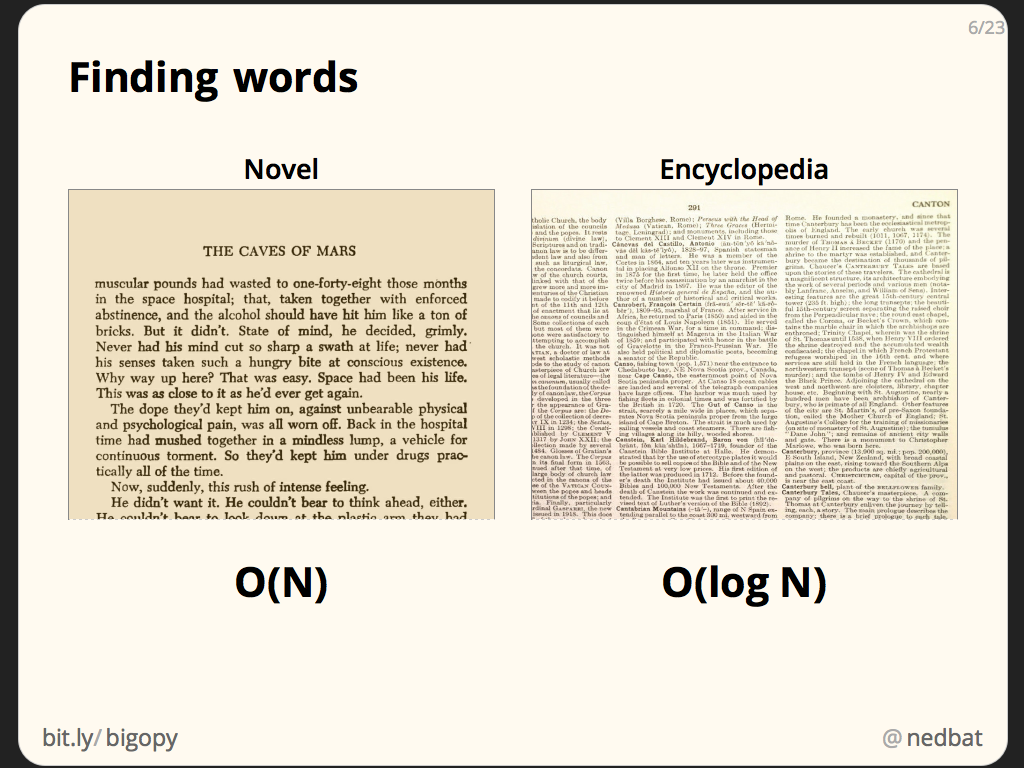Let’s consider another real-world example: finding words in a book. If I hand you a novel, and ask you to find the word “horse” in it, you would start at the beginning and read until you found it. This is another O(N) task. If you double the length of the novel, it could take you twice as long to find it.

Now I hand you another book: an encyclopedia. Finding the word “horse” is easier now. You open to the middle of the book, and see whether the desired word is before or after the word on the page. If it’s before, then you open to the middle of the left-hand side, and continue your divide-and-conquer strategy until you find the word.

In this case, doubling the size of the encyclopedia doesn’t double the time it takes. It just adds one more divide-in-half step to the work. This is known as a log-N task: the number of steps grows as the number of digits in N grows.

Counting beans and finding words are both examples of real-world tasks that have very different growth rates, depending on the details of the job. Big-O notation is a way to understand those growth rates. Understanding the algorithmic complexity of your code is important for writing efficient code that performs as you want.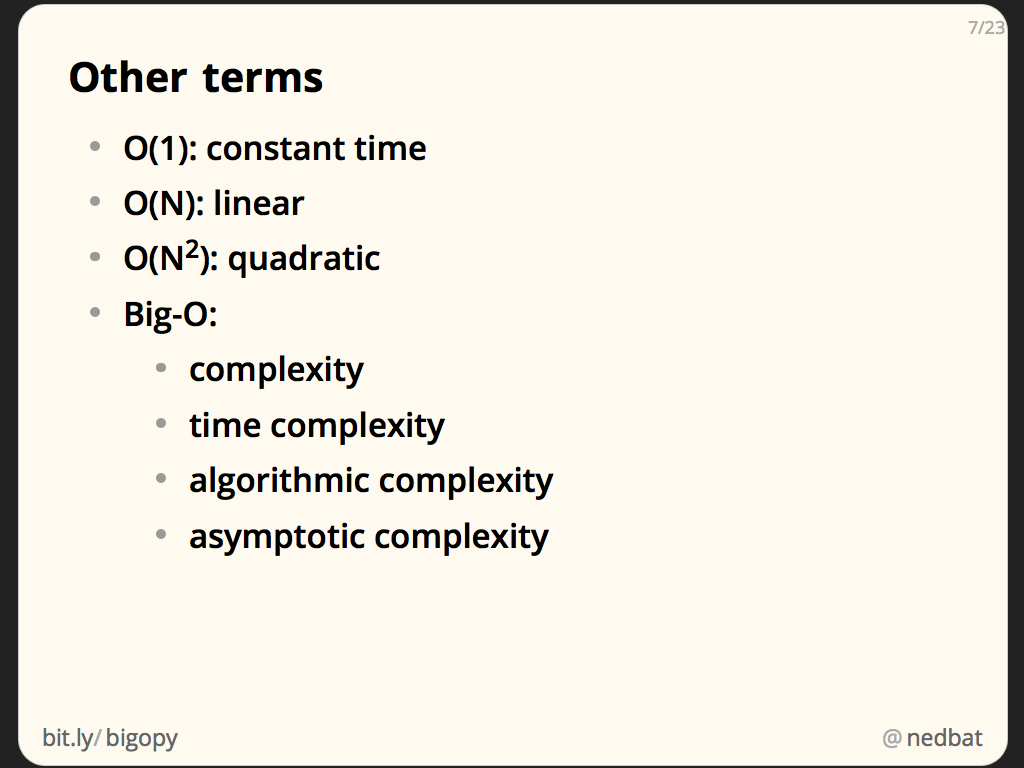A little more terminology to get out of the way. Some of the more common big-O characterizations have short-hand names. O(1) is sometimes called “constant time” or just “constant” because it doesn’t change as the data size changes. O(N) is often called “linear” because the time is a linear expression of the data size. And O(N2) is called “quadratic” because the time is a quadratic expression of the data size.

The whole idea of big-O is also known as “algorithmic complexity” or “algorithmic analysis.” Those sound complicated and fancy, but it’s all the same stuff. Sometimes the big-O characterization is called the “complexity” of an algorithm, or the “time complexity.”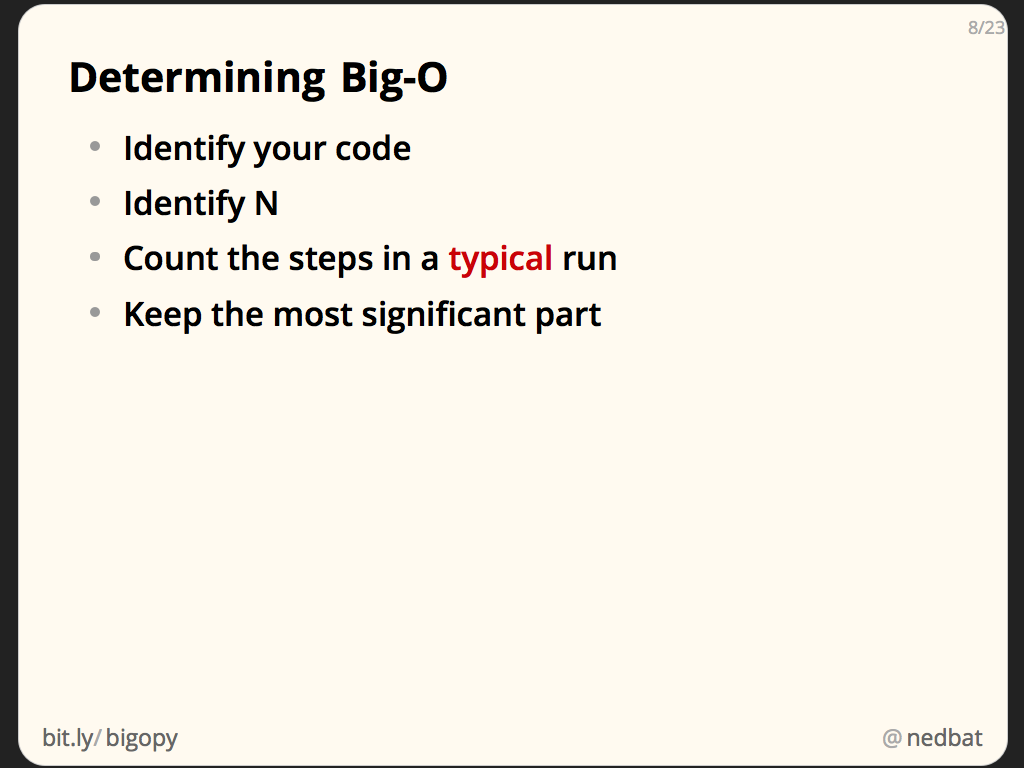OK, so how can you determine the Big-O complexity of a piece of code?

First, figure out what piece of code you are going to consider. This may seem obvious, but if you are unclear about the boundaries of the code, you may get an answer that is useless to you. Working in large systems, it’s easy to focus on too small a part of the code, or to try to think about too large a piece of the system.

Second, identify what N means. This will be the size of the data the function is working on. Usually, it will be fairly obvious. But sometimes, there will be more than one way the data can change size, and you need to figure out which one you are concerned with. N will be the size of that data.

Third is the real work: you read your code carefully, considering how many steps of work it will do in a typical execution run. Keep track of the number of steps, expressed in terms of N. “Typical” here means two things: you can consider typical real-world data, and also you should think about the average performance over many runs of the code.

Once you have a complete expression for the number of steps, you simplify it by throwing parts of it away. Big-O is all about how the run time changes as data gets larger and larger. So only the most significant terms need to be kept.

For example, if you counted 2N+1 steps, then you have an O(N) algorithm. There’s no point keeping the +1, because as N grows larger and larger, the +1 becomes less and less significant. Also, the 2 coefficient doesn’t matter: 2N grows the same as N does: if N doubles, then 2N doubles, and N doubles. So O(2N+1) is the same as O(N), and we keep the simpler form.

Notice there are no units involved here. We aren’t trying to calculate the actual running time of the code. We’re trying to understand how the running time changes as the data size changes.

About “typical”: big-O doesn’t by itself imply typical runs. You can also talk about the big-O of the worst case, which we’ll touch on later. But when people casually mention the big-O complexity of code, they usually mean the typical, or expected running time. If you are unsure which people mean, ask for clarification.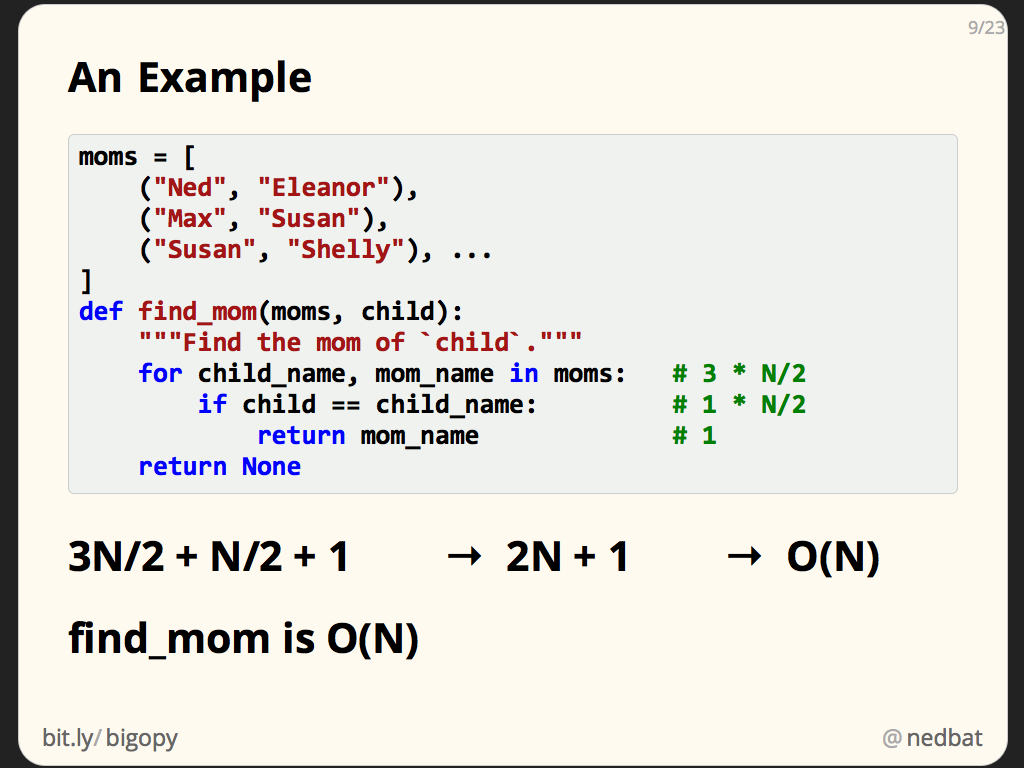Here’s a real example:

`moms = [    ("Ned", "Eleanor"),    ("Max", "Susan"),    ("Susan", "Shelly"), ...]def find_mom(moms, child):    """Find the mom of `child`."""    for child_name, mom_name in moms:        if child == child_name:            return mom_name    return None`

The find_mom function searches a list of tuples looking for a match on the first element of the tuple. In this case, N is the length of the list of tuples.

Since we don’t know where any given value is in the list, we don’t know whether we’ll find it at the beginning of the list, or near the end. So we guess that over many runs of the function, we’ll have to look at (on average) half the entries in the list.

In our average run, looping over the moms will happen N/2 times. Each time around, there’s one step to get the next element, and one step each to assign the tuple to child_name and mom_name. So the for-loop line gives us 3*N/2 steps.

The comparison is just one step, but since we loop N/2 times, it contributes another N/2 steps. The return statement happens only once, for 1 more step.

The grand total is 3N/2 + N/2 + 1, or 2N+1. Since we are only interested in the large-scale growth trends as N gets very large, we drop the +1, and the 2 coefficient, to get O(N).

Our analysis has shown us this is a linear algorithm: when the moms list doubles in size, we can expect find_mom to take twice as long.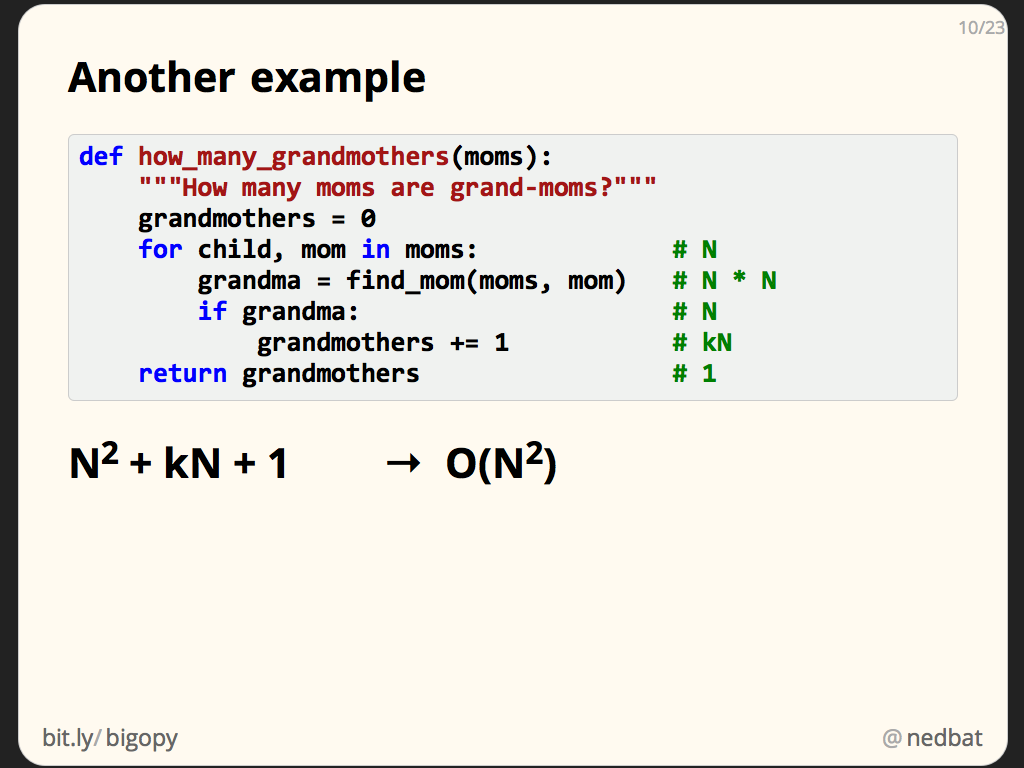Let’s consider another example. With our moms data structure, let’s consider this function to count how many of the entries in our list are grandmothers: the mom in the pair also appears as a child in another pair:

`def how_many_grandmothers(moms):    """How many moms are grand-moms?"""    grandmothers = 0    for child, mom in moms:        grandma = find_mom(moms, mom)        if grandma:            grandmothers += 1    return grandmothers`

We know that we are only going to keep the most significant component, and we don’t care about the coefficients, so we won’t bother to track them. Instead we’ll just note the exponent for each part of the code.

Here we’re going to loop over the entire moms list so our loop will execute N times. The for statement itself will contribute N steps.

The next line is the interesting one though: we call find_mom. We just did the analysis of find_mom, so we know it’s O(N). This line contributes N steps each time it’s executed, but it’s executed N times, once for each execution of the loop. So this line contributes N2 steps.

We can continue with the rest of the analysis, but note that we already have an N2 component, so N components or constant components will eventually be discarded, so we can skip collecting them.

Because the largest component is N2, the result is O(N2).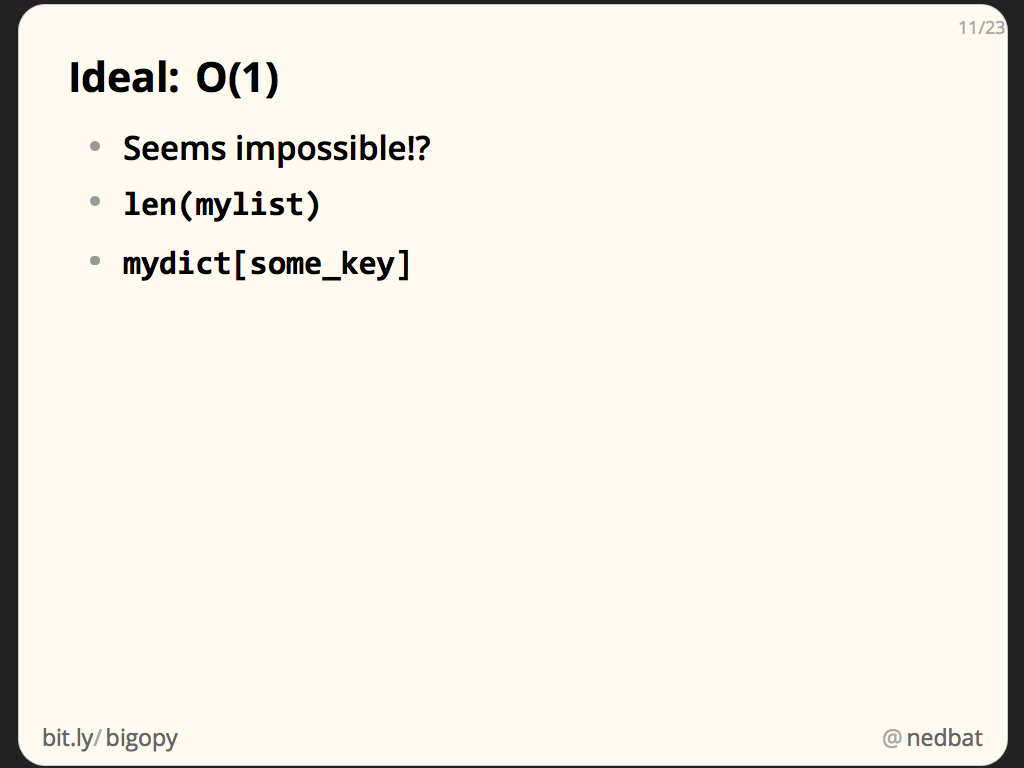The best possible complexity is constant-time: no matter how much data, it always takes the same amount of time. This seems kind of impossible. How can an algorithm take a fixed amount of time no matter how much data it has to work on?

We saw one example with the labelled jars of jelly beans. Python lists work the same way: getting the length of a Python list is an O(1) operation because the list stores its length outside of the actual data of the list, just as the bean jar has a count on the outside.

A more interesting example is looking up a key in a dict. In the typical case, looking up a key in a dict takes the same amount of time no matter how large the dict is. This is why dictionaries underpin every name lookup in Python: they are fast. I don’t have time to go into how this works, but it’s because dictionaries are a clever data structure called a hash table. Later we’ll talk about the worst-case scenarios, but for the typical (that is to say, almost every) case, dictionary access is O(1).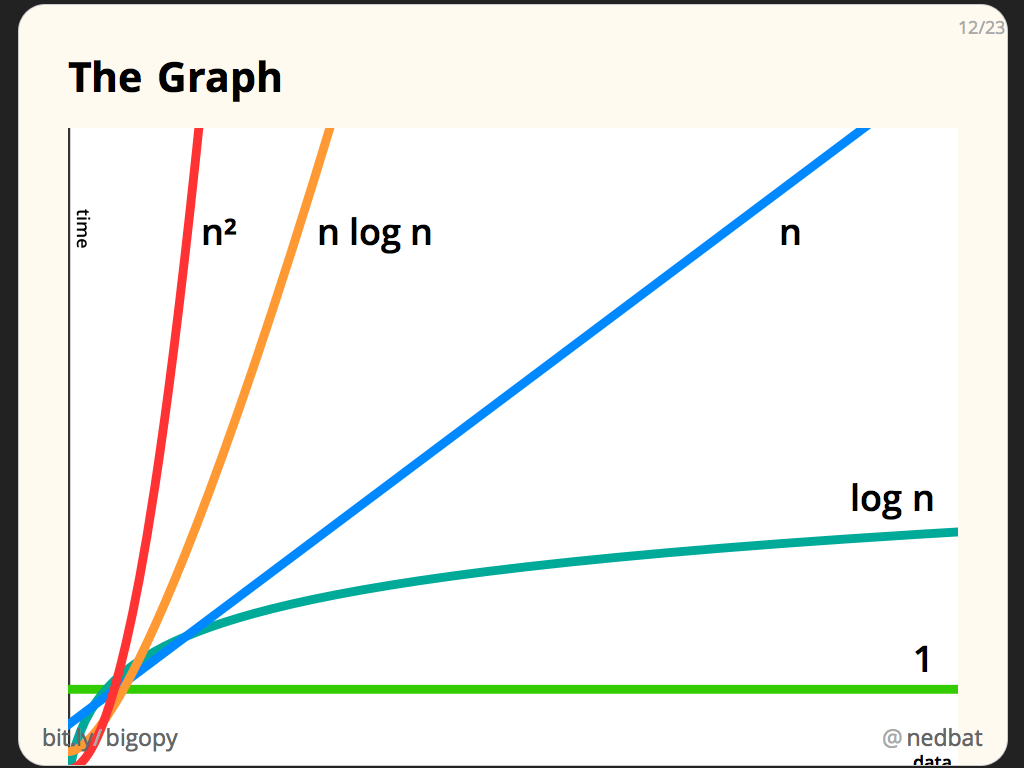No discussion of big-O notation would be complete without showing a graph of how various complexities grow. Here the x-axis is the growth of data, and the y-axis is running time. There are no units here, because as with all the rest of this discussion, we are only looking for a rough characterization of how the running time relates to the data size.

You can see here that N2 can get really bad really fast. If there’s one thing to take away from this entire talk, it’s “avoid N2“.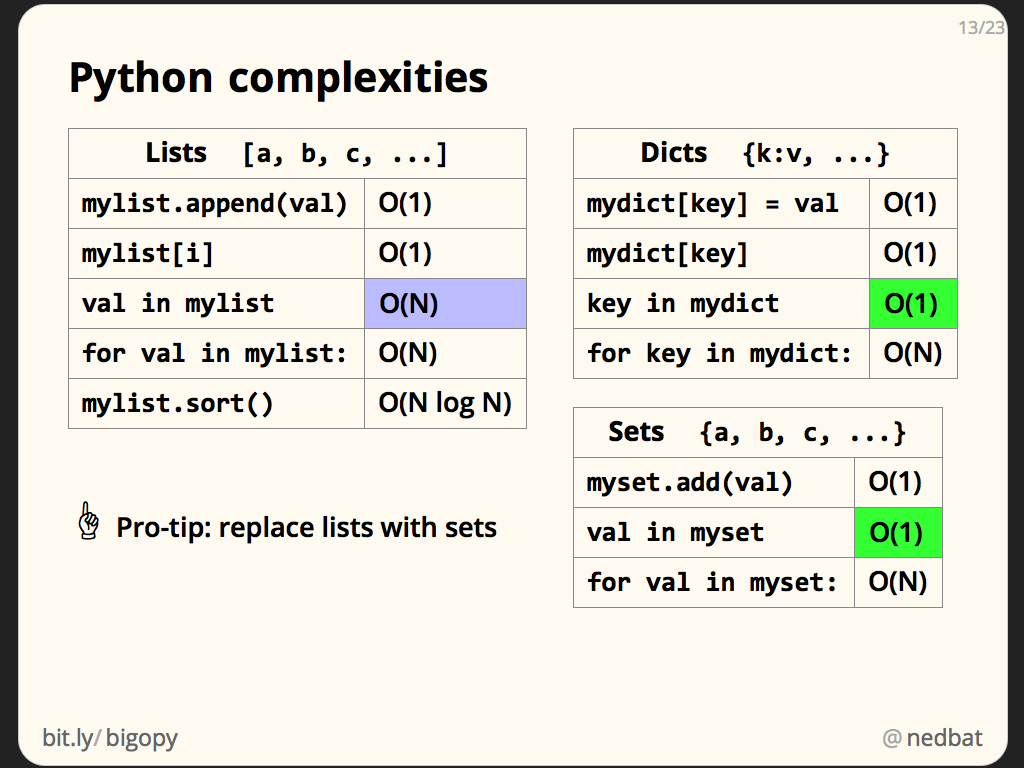To analyze your code, you have to understand the complexities of the Python primitives you’ll be using. These tables show the typical time complexity of common Python operations.

Many of the similar operations on list, dict, and set have the same complexities. A big difference is finding an element in each. In particular, finding an element in a list is O(N), while finding an element in a dict or set is O(1). This can make a huge difference in your program’s speed. If you can replace a list lookup with a set lookup, do it.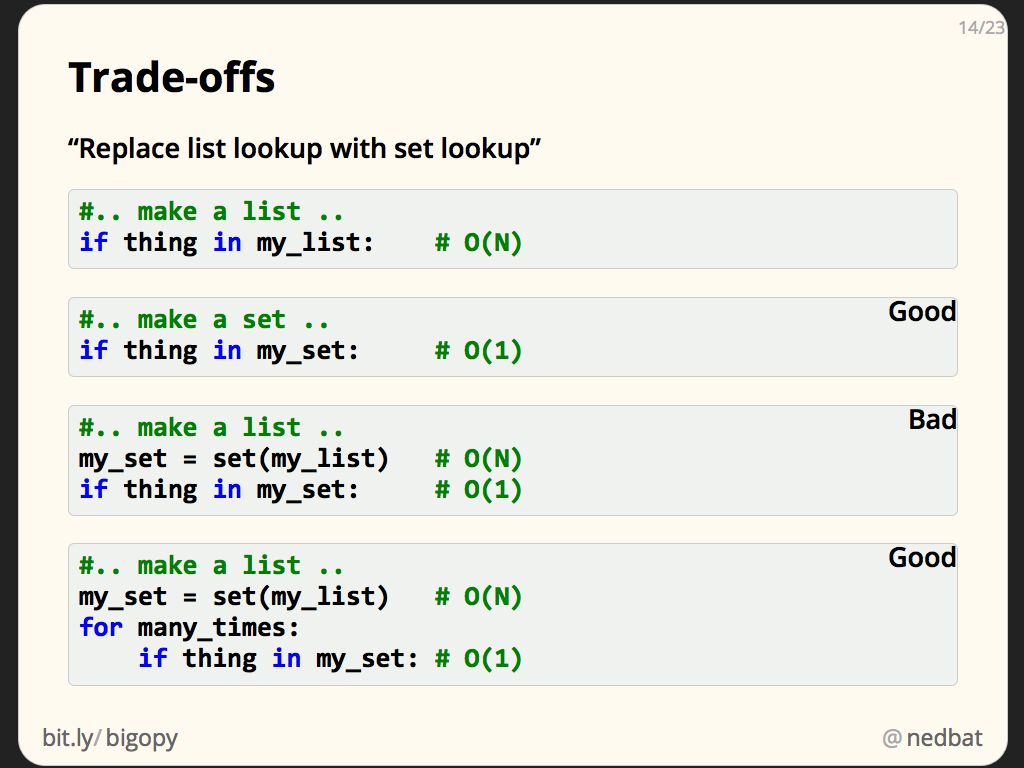Replacing a list lookup with a set lookup is good, but you have to be careful. The best way is to not create a list in the first place. Instead, create a set from the start.

The worst way to go is to have a list in hand, and then make a set from it, then do the lookup in the set. The set lookup is a fast constant-time lookup, but what did it cost to create the set? Creating a set from an iterable is an O(N) operation because every element of the iterable has to be considered.

Making a set just to perform one lookup is a waste of time. But it can be a good trade-off if you can make the set once and then use it for many lookups.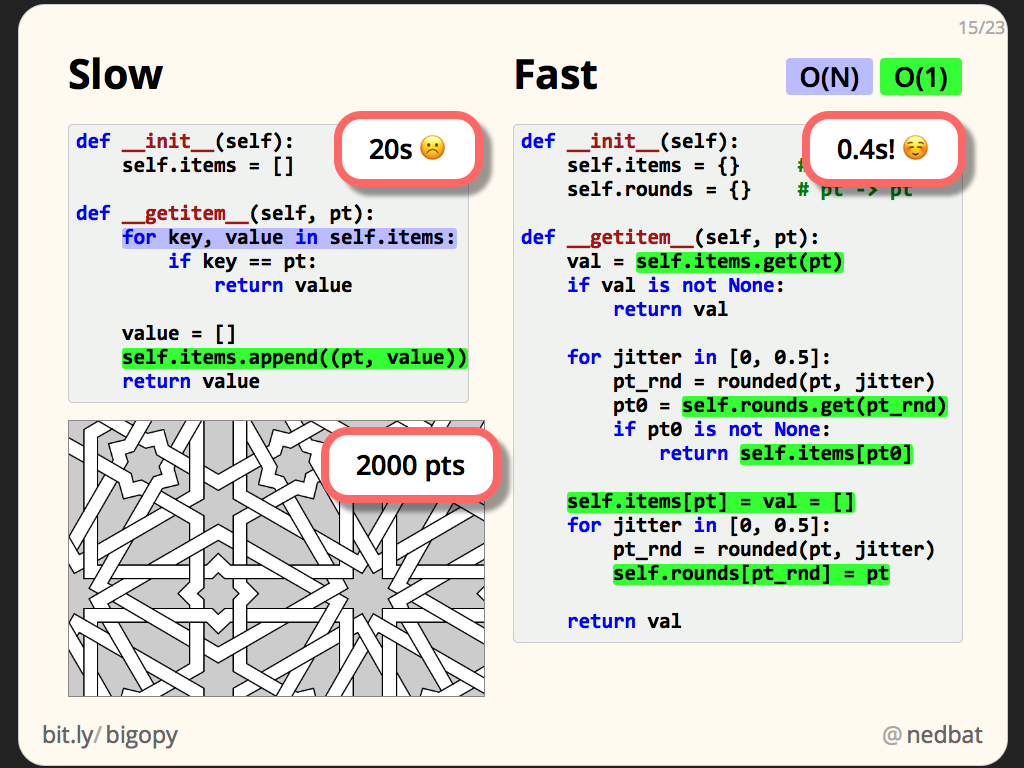Here’s an example of two implementations of a function. Don’t worry about the details of the code. Although the left one is shorter, and uses fewer data structures, it’s slower. If we look at the lines that deal with the data, we can see why. The left code has an O(N) line, while the right code only has O(1) lines.

This code operates on a collection of points. You can’t see it here, but in the real program this function is called once for each point in the collection, so the O(N) line in effect becomes an O(N²) line. The left code made the whole program O(N²), while the right code made it O(N).

Running with 2000 points, the N² code took 20 seconds. Switching to the O(N) code reduced the time to 0.4 seconds. You might not consider 2000 points to be a lot of data. But for that size data the difference between O(N) and O(N²) can be the difference between two thousand and four million. It can make a big difference.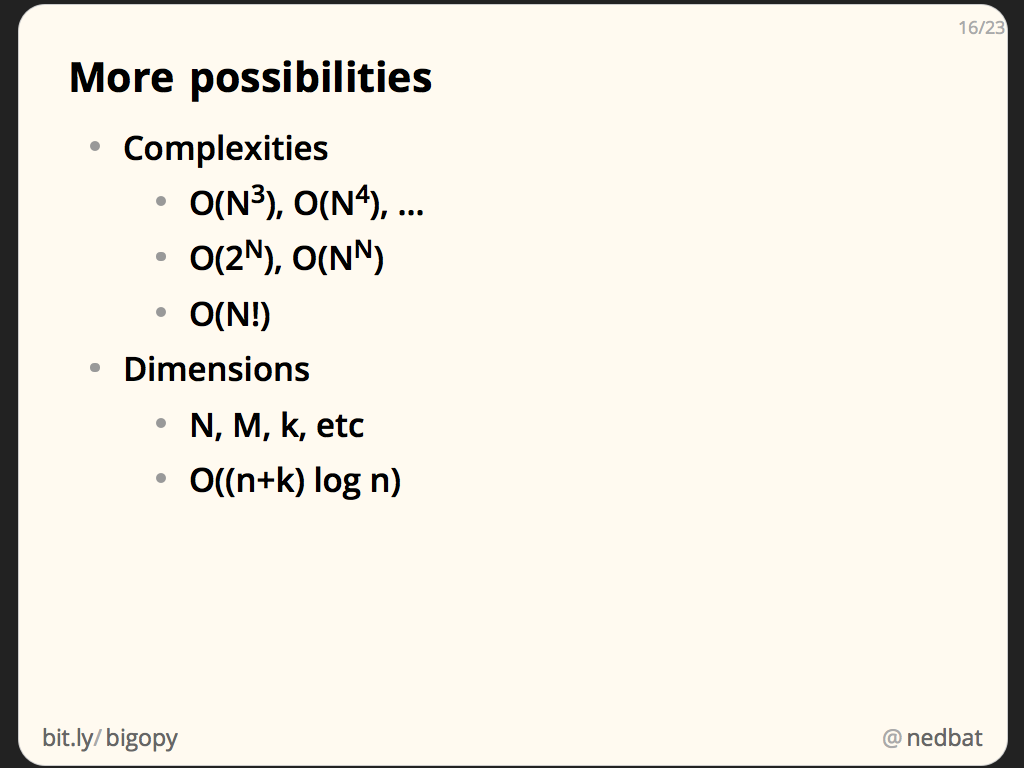The possibilities for algorithmic complexities go beyond the 1, N, N² that we’ve seen so far. There are all sorts of other forms that can occur, usually worse than the N² we’ve been demonizing here so far. Considering all possible combinations of N boolean values will give you O(2N). Operating on all permutations of N values will be O(N!).

You might also need more than one varying dimension. Sometimes your code will deal with data that can grow in more than one way. In that case, you use another variable, and describe the complexity in terms of both. As an example, a line intersection algorithm I used has a complexity of O((n+k) log n), where n is the number of lines, and k is the number of intersections.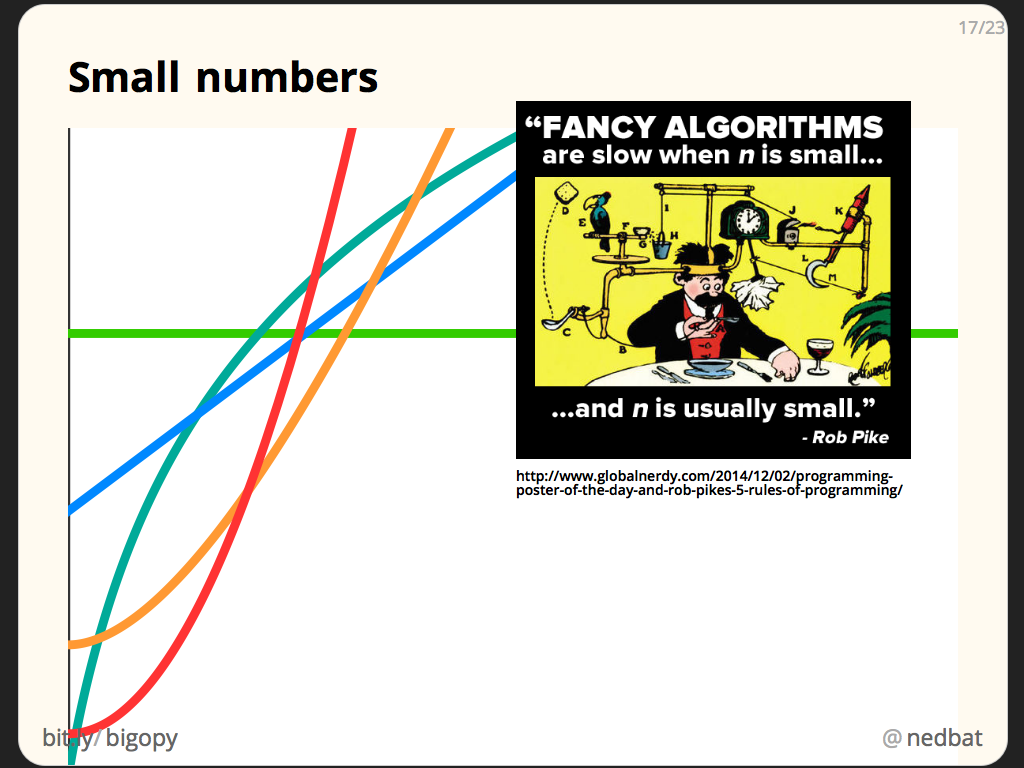Don’t fall into the trap of thinking that Big-O is everything though. What you really care about is the running time of your code on your actual expected data. An O(N²) algorithm might be faster than an O(N) algorithm for the range of data you’ll be using.

Here I’ve zoomed into the graph down near the small numbers. The curves are all scrambled up because the actual coefficients I used make the constant-time algorithm slower than the others for the very small numbers.

When we discarded the coefficients and smaller exponents, it was because as N grows very large, they don’t matter. But in actually running your code, those components are still there, and for small data, they may be more important than the larger ones we kept.

Don’t get fancy unless you have to. For small data the simplest algorithms, even if they have worse complexity, are best.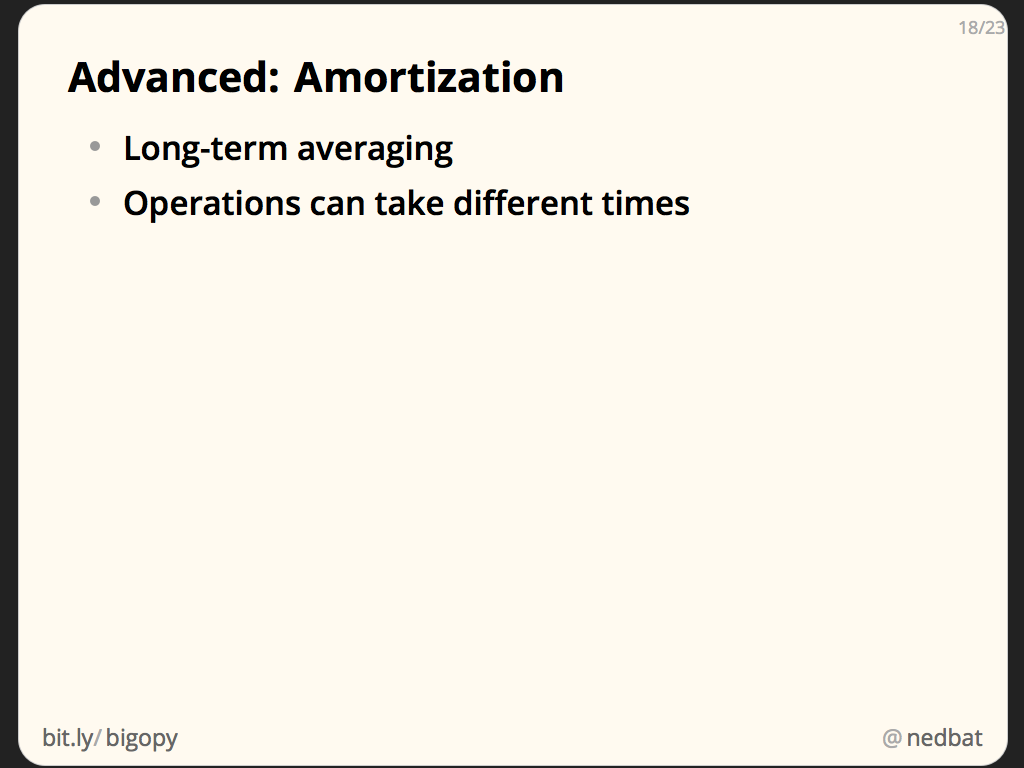Let’s touch quickly on some advanced pieces.

Big-O is about the long-term growth of an algorithm. That can involve averaging many operations. Individual operations may not all take the same amount of time. This is called amortization, which is a fancy word that means averaging.

For example, we say that appending to a list is O(1), but that doesn’t mean that every call to append() takes the same amount of time. In fact, most of the calls take the same short time, but some of the calls take longer, and those long calls get longer as the list gets longer. But they also get less frequent, so the average is a constant time.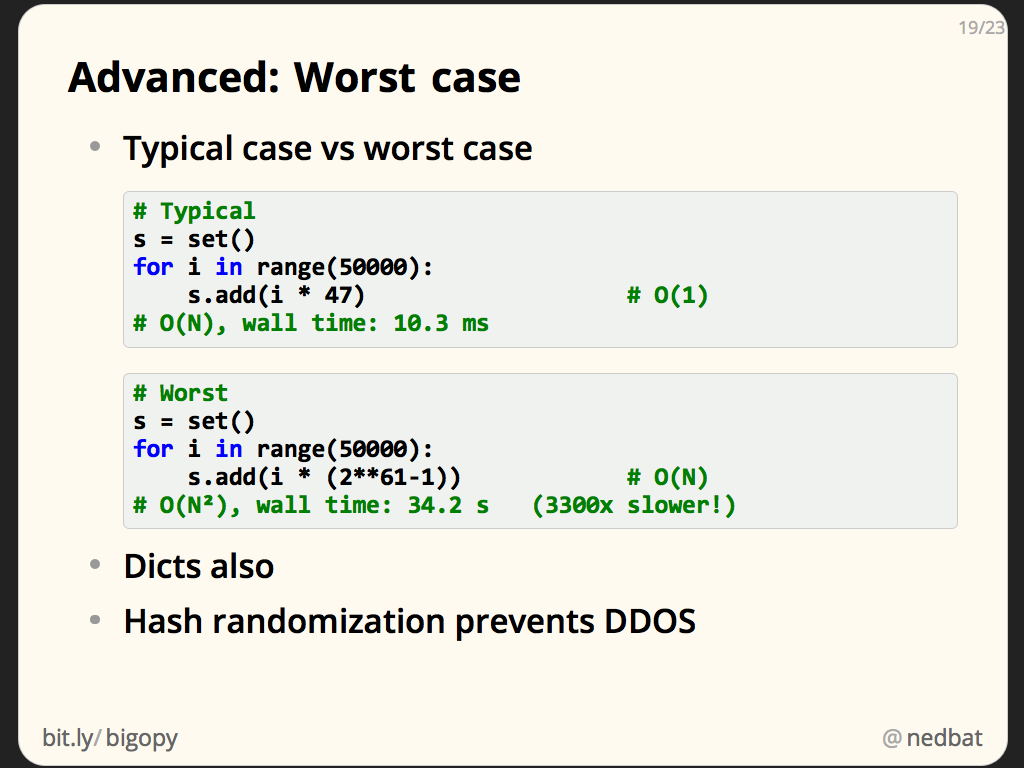So far all of this discussion has been about the typical, or expected time for operations. That is, the time the algorithm will take for a typical data set, averaged over many runs. Sometimes it’s also important to consider the worst case. How will the algorithm perform if given specially crafted data that will make it take as long as possible?

As an example, looking up a value in a set is typically O(1), which makes building a set an O(N) operation. But if you know how values are hashed, you can use a collection of values that all hash to the same value. This worst-case scenario makes the lookup O(N), which makes building the set O(N²).

Here are a typical case and a worst case:

`# Typicals = set()for i in range(50000):    s.add(i * 47)                   # O(1)# O(N), wall time: 10.3 ms`
`# Worsts = set()for i in range(50000):    s.add(i * (2**61-1))            # O(N)# O(N²), wall time: 34.2 s   (3300x slower!)`

Dictionaries have the same worst-case behavior. This was the basis for a denial-of-service attack against web servers. The defense against the attack was hash randomization, which prevented the attackers from knowing how strings would be hashed.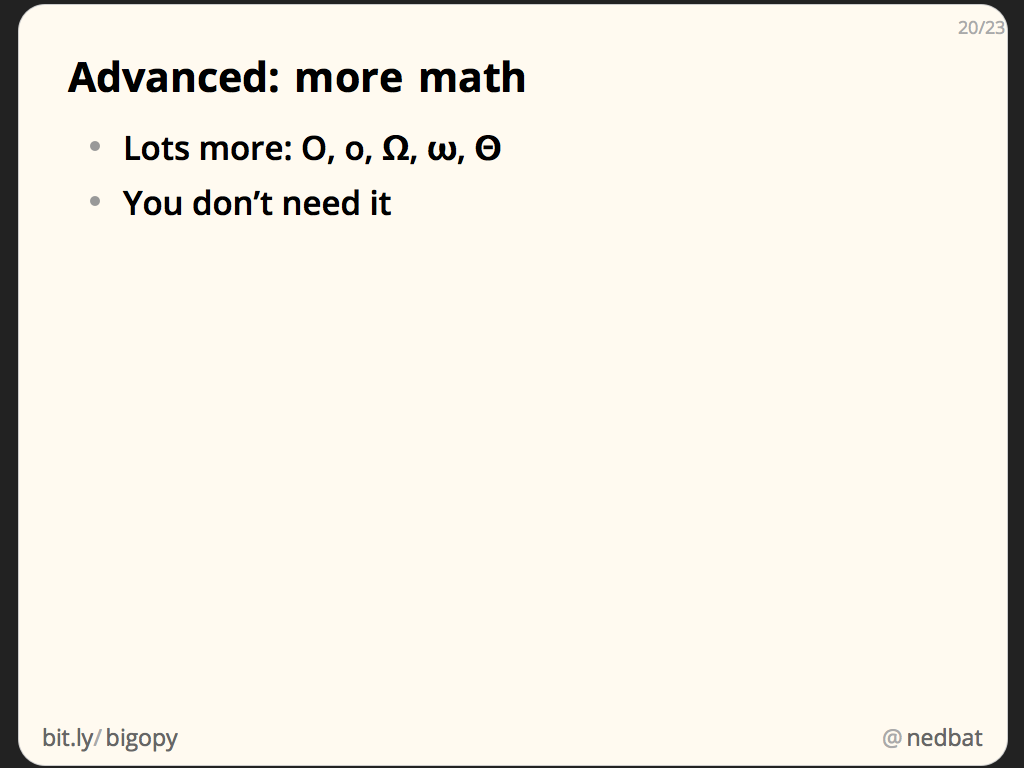Lastly, if you do more research into this, there is much more math involved, with more symbols. Mathematicians have used every letter that sounds like or looks like O to mean something for algorithmic analysis. Know that for day-to-day programming, you already have everything you need. If you enjoy the math, go for it, but don’t feel like you have to.If you do discuss this more, a word of warning: experts are not always nice. When I began thinking about this topic last fall, I wrote it up on my blog. A lot of people seemed to like it.

But one commenter was less pleased. While their tone could use some work, I appreciated the technical point they tried to make. I looked into it more and decided they were actually wrong about it. They didn’t agree.

But I got another blog post out of it...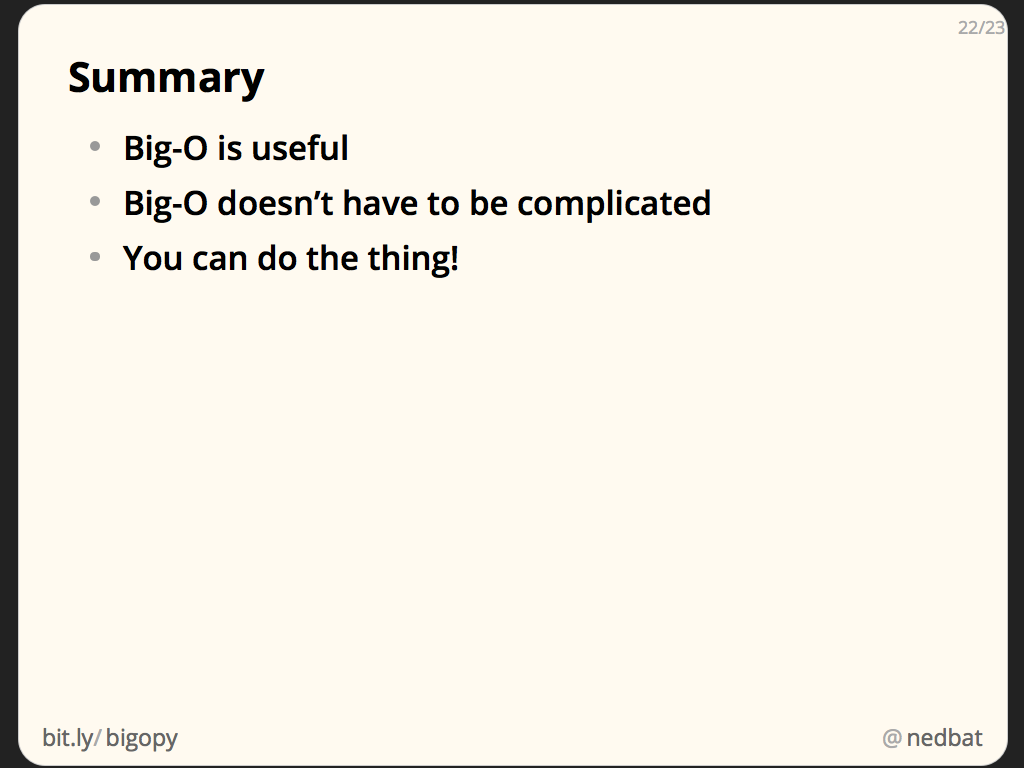I hope what you’ve gotten from this is that Big-O is useful, and doesn’t have to be complicated. Thanks.Brandon

Hi Ned,

when it comes to python I am a newbie and I am looking around the internet for great threads, articles or presentations like this one. So first of all thank you for sharing your knowledge.

What helped me the most were the slides #14, #15 and #19 - but the most valueble slide to me was #13. Do you have any further informations about functions/methods and their complexity or can you tell me how I can figure it out by my own?

Thank you,
Brandon@Brandon: I'm really glad this helped you. You make a good point about slide #13: it should have a link to https://wiki.python.org/moin/TimeComplexity, which is a more extensive summary of the time complexities of Python built-in operations.It's really perplexing. Despite being totally obvious that reading the jar label is not O(1) but O(log N), and despite my 3 comments to the contrary, you _still_ stubbornly insist it's O(1). Do you not understand the argument, or you just think the problem will go away if you ignore it?@Veky: Humans don't read words and numbers a character at a time. They take in the gestalt of the word. It may be that a long number will take longer to read than a short number, but it is not linear. People don't take 4 times longer to read the number "1000" than they do to read "1".

I feel comfortable in using O(1) for that first cartoon example to demonstrate the concept of a task having different complexity based on how the data is organized and accessed.Anurag Sharan

@Veky another great example of toxic experts.shubham

Hello Ned,

I watched your pyCon talk and I landed here from there with a specific doubt. In slide 19, where you talk about typical and worst case - the compute time for i*47 and i*(2**61-1) might be different but still the same for multiple rounds. I don't understand how the former makes the code O(N) whereas the later O(N^2).

You also mention something about hashing there which I don't understand in the context.

Please shed some light on this.

Thank you.Thanks for this! I have a lot of work to do to improve the performance of my Python code, but this is the closest I've gotten to grokking the concepts. I feel like I kind of have a mental box to put the details in now.

I'm reading the book below for the nitty-gritty details, it's pretty challenging so far. Do you have any recommendations on books or resources to wrap my non-mathy head around writing faster Python?

Python Algorithms: Mastering Basic Algorithms in the Python Language, Second Edition
by Magnus Lie Hetland@Veky, you think that 9000 takes longer to read than 371? No, of course it's quite the opposite. While rounder/simpler numbers are quicker for humans to read, that's something that increases and decreases with n (and not by a meaningful difference).

In any case, as we're talking about computer algorithms, the CPU will just copy the whole byte (or whatever data size) without considering what the number the bits represent. It is a constant time operation.Bruno Claro

Hi Ned, thank you for this great explanation. The O notation concept is new to me and i have a question. What about chunking data when data grows? if the data is always limited by a certain size limit, would this reduce the code slowing down? Would that be more important than the way the code is written?.

Thank you.@Bruno: chunking sounds like you are still processing all of the data, so it won't change the time complexity. It could be a good way to reduce the overall time, but that's a different matter